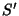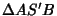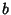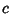## Isoperimetric PointThe pointwhich makes the Perimeters of the Triangles,, andequal. The isoperimetric point exists Iff the largest Angle of the triangle satisfiesor equivalentlywhere,, andare the side lengths of,is the Inradius, andis the Circumradius. The isoperimetric point is also the center of the outer Soddy Circle ofand has Triangle Center FunctionReferences

Kimberling, C. Central Points and Central Lines in the Plane of a Triangle.'' Math. Mag. 67, 163-187, 1994.

Kimberling, C. Isoperimetric Point and Equal Detour Point.'' http://cedar.evansville.edu/~ck6/tcenters/recent/isoper.html.

Kimberling, C. and Wagner, R. W. Problem E 3020 and Solution.'' Amer. Math. Monthly 93, 650-652, 1986.

Veldkamp, G. R. The Isoperimetric Point and the Point(s) of Equal Detour.'' Amer. Math. Monthly 92, 546-558, 1985.